Binomial Probability - "Exactly" MathBitsNotebook.com Terms of Use   Contact Person: Donna RobertsIn a binomial experiment there are two mutually exclusive  outcomes, often referred to as "success" and "failure".  If the probability of success is p, the probability of failure is 1 - p. Such an experiment whose outcome is random and can be either of two possibilities, "success" or "failure", is called a Bernoulli trial, after Swiss mathematician Jacob Bernoulli (1654 - 1705).Examples of Bernoulli trials: • flipping a coin - heads is success, tails is failure • rolling a die - 3 is success, anything else is failure • voting - votes for candidate A is success, anything else is failure • determining eye color - green eyes is success, anything else is failure • spraying crops - the insects are killed is success, anything else is failureWhen computing a binomial probability, it is necessary to calculate and multiply three separate factors:

1.  the number of ways to select exactly r successes,

2.  the probability of success (p) raised to the r power,

3.  the probability of failure (q) raised to the (n - r) power.

 The probability of an event, p, occurring exactly r times: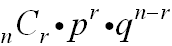n = number of trials  r = number of specific events you wish to obtain  p = probability that the event will occur  q = probability that the event will not occur (q = 1 - p, the complement of the event)
If we use an alternate notation for combination, and express the complement value q as (1 - p),
we have an alternate formula for
binomial probability.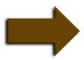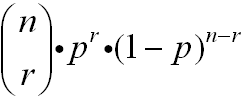Alternative formula formThe graphing of all possible binomial probabilities related to an event creates a binomial distribution.  Consider the following distributions of tossing a fair coin:

 Two Toss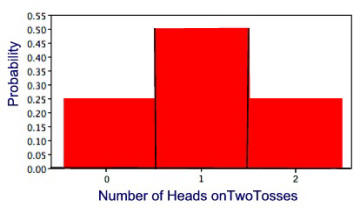Four Toss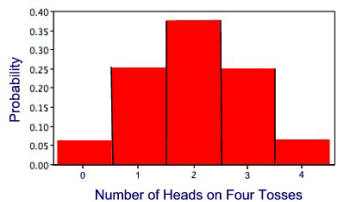In the following examples, answers will be rounded to 3 decimal places.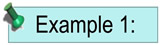A test consists of 10 multiple choice questions with five choices for each question.  As an experiment, you GUESS on each and every answer without even reading the questions.  What is the probability of getting exactly 6 questions correct on this test?Solution: n = 10 r = 6 n – r = 4 p = 0.20 = probability of guessing the correct answer on a question q = 1 - p = 0.80 = probability of not guessing the correct answer on a question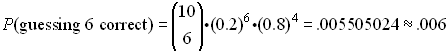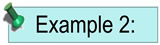When rolling a die 100 times, what is the probability of rolling a "4" exactly 25 times?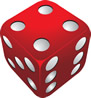Solution: n = 100 r = 25 n – r = 75 p = 1/6 = probability of rolling a "4" q = 1 - p = 5/6 = probability of not rolling a "4"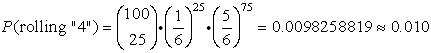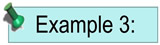At a certain intersection, the light for eastbound traffic is red for 15 seconds, yellow for 5 seconds, and green for 30 seconds.  Find the probability that out of the next eight eastbound cars that arrive randomly at the light, exactly three will be stopped by a red light.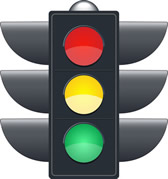Solution: n = 8 r = 3 n – r = 5 p = 15/50 = probability of a red light q = 1 - p = 35/50 = probability of not a red light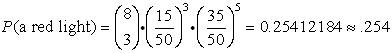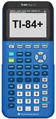For working with Bernoulli Trials on your calculator, click here.